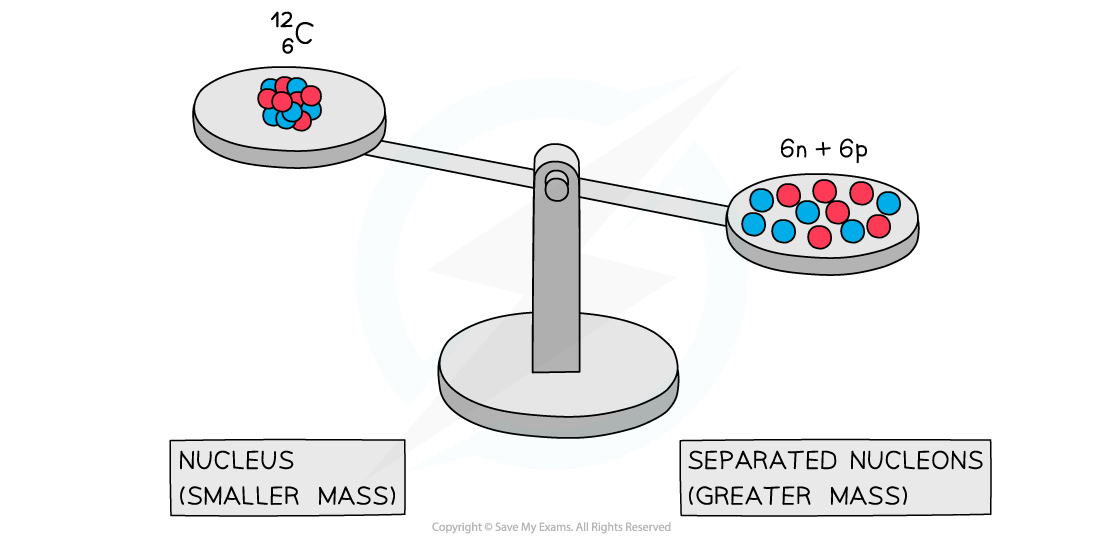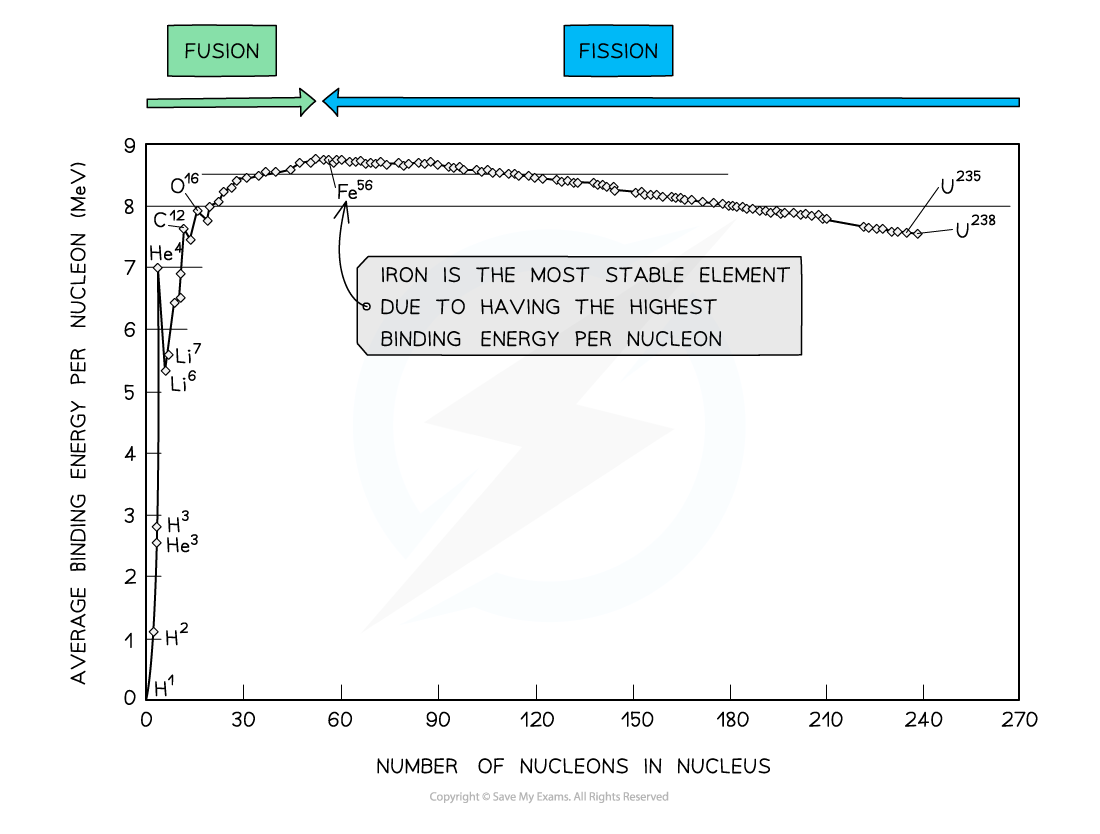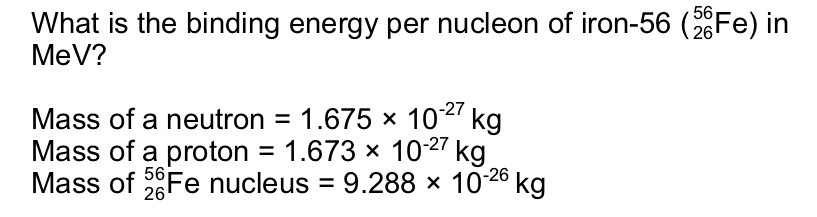# CIE A Level Physics复习笔记23.1.3 Mass Defect & Binding Energy

### Mass Defect & Binding Energy

• Experiments into nuclear structure have found that the total mass of a nucleus is less than the sum of the masses of its constituent nucleons
• This difference in mass is known as the mass defect
• Mass defect is defined as:

The difference between an atom's mass and the sum of the masses of its protons and neutrons

• The mass defect Δm of a nucleus can be calculated using:

Δm = Zmp + (A – Z)mn – mtotal

• Where:
• Z = proton number
• A = nucleon number
• mp = mass of a proton (kg)
• mn = mass of a neutron (kg)
• mtotal = measured mass of the nucleus (kg)A system of separated nucleons has a greater mass than a system of bound nucleons

• Due to the equivalence of mass and energy, this decrease in mass implies that energy is released in the process
• Since nuclei are made up of neutrons and protons, there are forces of repulsion between the positive protons
• Therefore, it takes energy, ie. the binding energy, to hold nucleons together as a nucleus
• Binding energy is defined as:

The energy required to break a nucleus into its constituent protons and neutrons

• Energy and mass are proportional, so, the total energy of a nucleus is less than the sum of the energies of its constituent nucleons
• The formation of a nucleus from a system of isolated protons and neutrons is therefore an exothermic reaction - meaning that it releases energy
• This can be calculated using the equation:

E = Δmc2

#### Exam Tip

Avoid describing the binding energy as the energy stored in the nucleus – this is not correct – it is energy that must be put into the nucleus to pull it apart.

### Binding Energy per Nucleon

• In order to compare nuclear stability, it is more useful to look at the binding energy per nucleon
• The binding energy per nucleon is defined as:

The binding energy of a nucleus divided by the number of nucleons in the nucleus

• A higher binding energy per nucleon indicates a higher stability
• In other words, it requires more energy to pull the nucleus apart
• Iron (A = 56) has the highest binding energy per nucleon, which makes it the most stable of all the elementsBy plotting a graph of binding energy per nucleon against nucleon number, the stability of elements can be inferred

#### Key Features of the Graph

• At low values of A:
• Nuclei tend to have a lower binding energy per nucleon, hence, they are generally less stable
• This means the lightest elements have weaker electrostatic forces and are the most likely to undergo fusion
• Helium (4He), carbon (12C) and oxygen (16O) do not fit the trend
• Helium-4 is a particularly stable nucleus hence it has a high binding energy per nucleon
• Carbon-12 and oxygen-16 can be considered to be three and four helium nuclei, respectively, bound together
• At high values of A:
• The general binding energy per nucleon is high and gradually decreases with A
• This means the heaviest elements are the most unstable and likely to undergo fission

#### Worked ExampleStep 1:            Calculate the mass defect

Number of protons, Z = 26

Number of neutrons, A – Z = 56 – 26 = 30

Mass defect, Δm = Zmp + (A – Z)mn – mtotal

Δm = (26 × 1.673 × 10-27) + (30 × 1.675 × 10-27) – (9.288 × 10-26)

Δm = 8.680 × 10-28 kg

Step 2:            Calculate the binding energy of the nucleus

Binding energy, E = Δmc2

E = (8.680 × 10-28) × (3.00 × 108)2 = 7.812 × 10-11 J

Step 3:            Calculate the binding energy per nucleonStep 4:            Convert to MeV

J → eV: divide by 1.6 × 10-19

eV → MeV: divide by 106#### Exam Tip

Checklist on what to include (and what not to include) in an exam question asking you to draw a graph of binding energy per nucleon against nucleon number:

• You will be expected to draw the best fit curve AND a cross to show the anomaly that is helium
• Do not begin your curve at A = 0, this is not a nucleus!
• Make sure to correctly label both axes AND units for binding energy per nucleon
• You will be expected to include numbers on the axes, mainly at the peak to show the position of iron (56Fe)